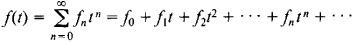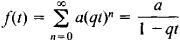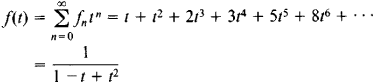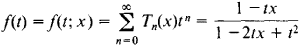# Generating Function

(redirected from Ordinary generating functions)

## generating function

[′jen·ə‚rād·iŋ ‚fəŋk·shən]
(mathematics)
A function g (x, y) corresponding to a family of orthogonal polynomials ƒ0(x), ƒ1(x),…, where a Taylor series expansion of g (x, y) in powers of y will have the polynomial ƒn (x) as the coefficient for the term y n .
A function, g (y), corresponding to a sequence a0, a1, ⋯) where g (y) = a0+ a1 y + a2 y 2+ …. Also known as ordinary generating function.
McGraw-Hill Dictionary of Scientific & Technical Terms, 6E, Copyright © 2003 by The McGraw-Hill Companies, Inc.
The following article is from The Great Soviet Encyclopedia (1979). It might be outdated or ideologically biased.

## Generating Function

A generating function of the sequence f0, f1,..., fn is the functionassuming that this power series converges for at least one nonzero value of t.

The sequence f0, f1,..., fn,… can be a sequence of numbers or of functions. In the latter case, the generating function depends not only on t but also on the arguments of the functions fn. For example, if fn = aqn, where a and q are constants, the generating function isIf the fn are Fibonacci numbers—that is, if f0 = 0, f1 = 1, fn+2 = fn+1 + fn—we haveIf fn = Tn (x) are Chebyshev polynomials—that is, if T0(x) = 1

and Tn (x) = cos (n arc cos x)—thenKnowledge of the generating function of a sequence often makes it easier to study the properties of the sequence. Generating functions are used in probability theory, in the theory of functions, and in the theory of invariants in algebra. Methods involving generating functions were first applied by P. Laplace to solve certain problems in probability theory.

### REFERENCES

Feller, W. Vvedenie v teoriiu veroiatnostei i eeprilozheniia, 2nd ed., vols. 1–2. Moscow, 1967. (Translated from English.)
Natanson, I. P. Konstruktivnaia teoriia funktsii. Moscow-Leningrad, 1949.